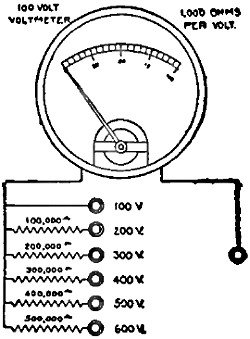# Determining Meter Shunts and MultipliersMay 1931 Radio-Craft

 May 1931 Radio-Craft[Table of Contents] Wax nostalgic about and learn from the history of early electronics. See articles from Radio-Craft, published 1929 - 1953. All copyrights are hereby acknowledged.

Analog and most digital multimeters rely on precision resistors for scaling the input voltage or current to keep it within the safe operational range of the meter movement or analog-to-digital converter circuit. Resistance value selection is a relatively simple matter of series and/or parallel combinations and their resulting divisions of voltages and/or currents. When this article appeared in 1931, the whole concept of electrical circuit design was entirely new to most people, including shunts and multipliers for meter scales. There is a nice table of resistor values provided, but I was a little disappointed to find that not a single equation is given for calculating custom values.

## Determining Meter Shunts and Multipliers

International Rectifier Company

Investment in a milliammeter or microammeter may be made to pay greater dividends, in the form of diversified applica­tions, by conversion to a multi-range volt­meter, Likewise, the usual voltmeter can be readily converted to a multi-range volt­meter.Fig. 1 - The ordinary 0-1 milliammeter, as shown, with the series resistors becomes a multi-scale voltmeter. The resistor values should, theoretically, be lessened by the resistance of the meter's moving element but, except on the 1-volt scale, this would make too trifling of a difference to be read.Fig. 2 - The same principle is applied to a standard voltmeters, except that the first series resistors is already provided.

The connections shown in Fig. 1 are intended for the conversion of microammeters and milliammeters into voltmeters. Those shown in Fig. 2 are for the conversion of a 100-volt, high-resistance meter to a multi-range voltmeter, by means of a number of precision wire-wound resistors which serve as voltmeter multipliers. In using resistors of this type as voltmeter multipliers, it is essential to know the internal resistance of the voltmeter, expressed in ohms per volt. Then multiply the full-scale reading of the voltmeter by the number of ohms per volt; in­sert a similar resistance value in series with the instrument, and the added resistor will double the effective scale reading.

The moving element of voltmeters may be used, in conjunction with a multiplier, to obtain lower ranges than those for which the meter was originally made, with a great saving over the cost of a new meter. The new multiplier or multipliers must be directly connected to the moving-element leads.

The resistance of the multiplier can be computed by the following formula: multiply the ohms per volt by the full-scale deflection; then the product by the desired multiplier ratio; and subtract the resistance of the moving-element. But since the re­sistance of most moving-elements is low (say 40 ohms to 0.1 ohm) it may be neglected except when the computed multiplier resistance is only fifty (or fewer) times the order of magnitude of the resist­ance of the moving-element.

The accompanying chart gives the total resistance required to change microammeters and milliammeters into instruments for accurately measuring voltage, Since the resistance of most microammeters and milliammeters is very low, that is, 40 ohms or much less, these values may be used for the multiplying resistors. The error in this assumption is the resistance of the instrument divided by the resistance given in the table; which, in most cases, is very much less than the error in most calibrations. The maximum error, other than the above, is the sum of the error of the moving-element and the error in the resistance value used. A moving element that is accurate to 2 percent would never become more accurate, no matter how accurate the multiplier was made. On the other hand, if a high degree of accuracy is wanted and the moving element was designed for that type of work, a very accurate multiplier must be used. If closer accuracies that 1 percent are required, resistors must be better than 1/2 percent.

Fortunately, wire-wound resistors of an accuracy of 1 percent and closer are now available commercially, as contrasted with the wider tolerances of resistors of the past. Furthermore, these resistor are thoroughly seasoned. These perfected wire-wound resistors now make it possible to convert meters into multi-range instruments with every assurance of accurate readings.December 22 16, 2014About RF CafeCopyright: 1996 - 2024Webmaster:    Kirt Blattenberger,    BSEE - KB3UON RF Cafe began life in 1996 as "RF Tools" in an AOL screen name web space totaling 2 MB. Its primary purpose was to provide me with ready access to commonly needed formulas and reference material while performing my work as an RF system and circuit design engineer. The Internet was still largely an unknown entity at the time and not much was available in the form of WYSIWYG ... All trademarks, copyrights, patents, and other rights of ownership to images and text used on the RF Cafe website are hereby acknowledged. My Hobby Website:  AirplanesAndRockets.com